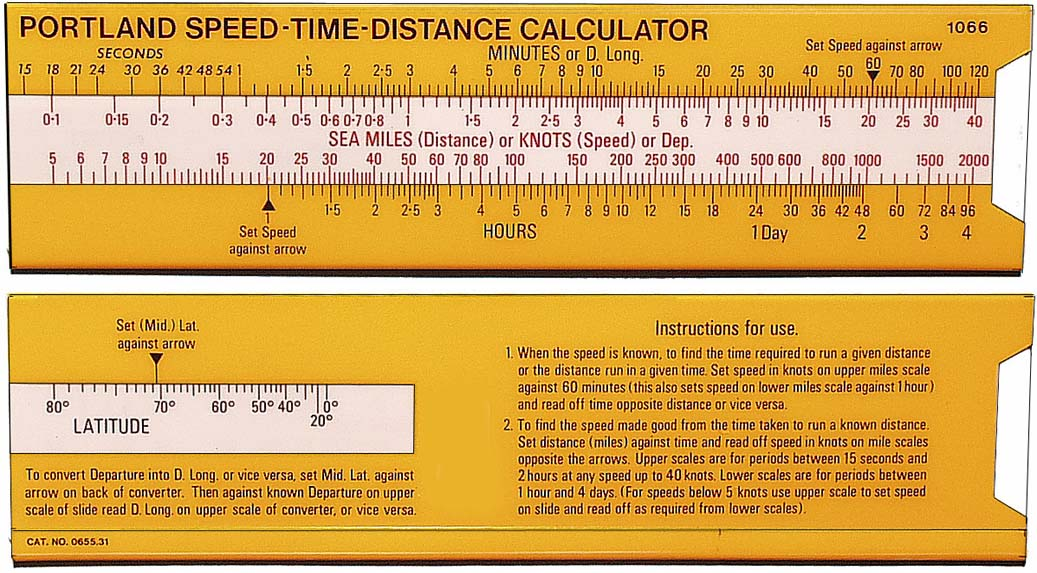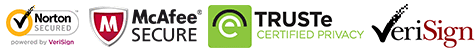# Speed / Time / Distance Calculatoror 4 interest free payments of \$4.98

Regular price \$19.95

Available: In stock
Speed - Time - Distance Calculator A simple-to-use slide rule device for calculating the third variable given the other two (distance covered, given speed and elapsed time).
QuantitySpeed - Time - Distance Calculator

A simple-to-use slide rule device for calculating the third variable given the other two (distance covered, given speed and elapsed time).

Vendor: bosunsboat N880 0.2 kg